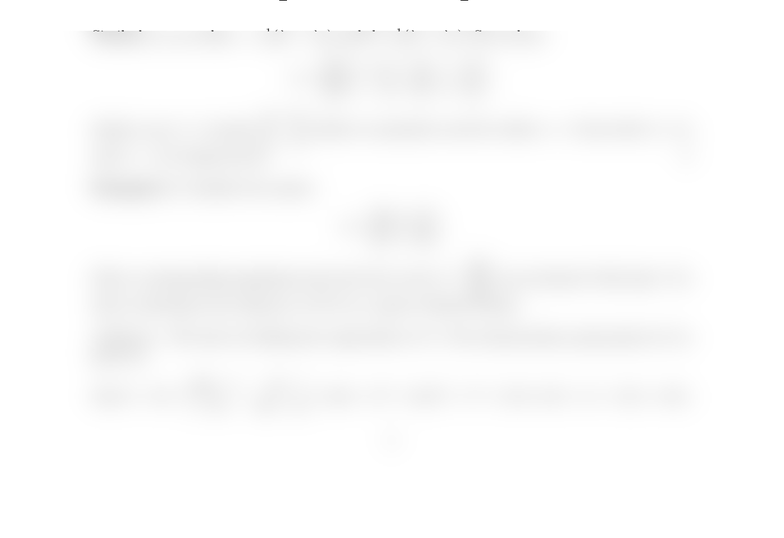Study Guides (380,000)
US (220,000)
UCLA (3,000)
MATH (300)
All (20)
Final

MATH 33AH Lecture Notes - Lecture 18: Eigenvalues And EigenvectorsExam

Department
Mathematics
Course Code
MATH 33AH
Professor
All
Study Guide
Final

This preview shows half of the first page. to view the full 2 pages of the document.Math 33A Week 8 Examples
Austin Christian
November 15 and 17, 2016
Example 1. Let λ1and λ2be real numbers and ﬁnd the unique 2 ×2 matrix which has
~e1+~e2and ~e1~e2as eigenvectors, with corresponding eigenvalues λ1and λ2, respectively.
(Solution) Denote the desired matrix by Aand write
A=a b
c d.
The eigenvector conditions given above tell us that
A1
1=λ1
λ1and A1
1=λ2
λ2,
so we have a+b
c+d=λ1
λ1and Aab
cd=λ2
λ2,
Because a+b=λ1and ab=λ2, we conclude that
a=1
2(λ1+λ2) and b=1
2(λ1λ2).
Similarly, we see that c=1
2(λ1λ2) and d=1
2(λ1+λ2). So we have
A=1
2(λ1+λ2)1
2(λ1λ2)
1
2(λ1λ2)1
2(λ1+λ2).
Indeed, any 2 ×2 matrix a b
c dwhich is symmetric and for which a=dhas both ~e1+~e2
and ~e1~e2of eigenvectors.
Example 2. Consider the matrix
A=0.6 0.4
0.4 0.6.
Find a corresponding eigenbasis and write the vector ~v =6
2as an element f this basis. Use
this to determine the behavior of An~v as ngrows without bound.
(Solution) We start by nding the eigenvalues of A. The characteristic polynomial of Ais
given by
det(AλI) =
0.6λ0.4
0.4 0.6λ
= (0.6λ)2(0.4)2=λ21.2λ+ 0.2 = (λ1)(λ0.2).
1